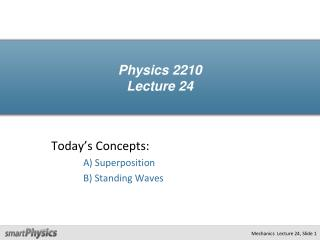DownloadDownload PresentationPhysics 2210 Lecture 24

# Physics 2210 Lecture 24

Télécharger la présentation## Physics 2210 Lecture 24

- - - - - - - - - - - - - - - - - - - - - - - - - - - E N D - - - - - - - - - - - - - - - - - - - - - - - - - - -
##### Presentation Transcript

1. Physics 2210Lecture 24 Today’s Concepts: A) Superposition B) Standing Waves

2. The Wave Equation

3. CheckPoint y v Case A x y Case B x v Suppose a pulse in Case A described by the function y(x,t) = P(x-vt). Which of the following functions described the pulse in Case B? A) y(x,t)=P(x +vt) B) y(x,t)= -P(x +vt) C) y(x,t) = -P(x -vt)

4. Superposition Q: What happens when two waves “collide?” A: They ADD together! Movie (super_pulse) Movie (super_pulse2)

5. Superposition A(ω1t) B(ω2t) C(t)= A(t)+ B(t) The wave equation we derived last time is linear. (It has no terms where the variables x,tare squared.) For linear equations, if we have two separate solutions, A and B, then A + B is also a solution! ω2 > ω1 DESTRUCTIVEINTERFERENCE CONSTRUCTIVEINTERFERENCE

6. Beats Can we predict this pattern mathematically? Of course! Just add two cosines and remember the identity: where and cos(ωLt) cos(ωHt) Demo

7. Standing Waves What happens when two waves having the same frequency but moving in the opposite direction meet? Wave 1 Wave 1 Wave 1 Wave 2 Wave 2 Wave 2 zero (Nodes) Movie (super) Superposition Mechanics Lecture 24, Slide 9

8. How it Works Stationary wave Changingamplitude How to make it: Demo

9. CheckPoint Suppose the strings on your guitar are 24” long as shown. The frets are the places along the neck where you can put your finger to make the wavelength shorter, and appear as horizontal white lines on the picture. When no frets are being pushed the frequency of the highest string is 4 times higher than the frequency of the lowest string. Is it possible to play the lowest string with your finger on any of the frets shown and hear the same frequency as the highest string? A) Yes B) No 24 20 frets 16 12 8 4

10. ACT The highest string vibrates with a frequency that is 4 times that of the lowest string. Compare the speed of a wave on the high string and the low string: A) vhigh = vlow*2 B) vhigh = vlow*4 C) vhigh = vlow*16 vhigh vlow

11. ACT The speed of a wave on the high string is 4 times the speed on the lowest string. If the tensions are the same, how does the mass per unit length of the strings compare: A) mlow =mhigh*2 B) mlow = mhigh*4 C) mlow = mhigh*16 Recall vhigh vlow

12. ACT Two cylinders have the same length and are made from the same material. If the mass of the bigger one is 16 times the mass of the smaller one, how do their diameters compare? L A) dlow =dhigh*2 B) dlow = dhigh*4 C) dlow = dhigh*16 dsmall dbig

13. CheckPoint dhigh dlow The 6 strings on a guitar all have about the same length and are stretched with about the same tension. The highest string vibrates with a frequency that is 4 times that of the lowest string. If the strings are made of the same material, how would you expect the diameters of the lowest and highest strings to compare? A) dlow =dhigh*2 B) dlow = dhigh*4 C) dlow = dhigh*16